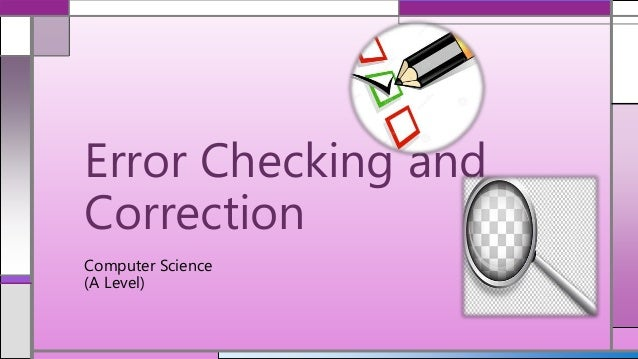Successfully reported this slideshow.Upcoming SlideShare
×

Error Checking and Correction (Parity Bit, Majority Voting, Check Digit)

4,025 views

Published on

Computer Science - Error Checking and Correction
This includes parity bit, majority voting and check digit which are all explained with their rules and more information.

Published in: Technology
• Full Name
Comment goes here.

Are you sure you want to Yes No• Be the first to comment

Error Checking and Correction (Parity Bit, Majority Voting, Check Digit)

1. 1. Computer Science (A Level) Error Checking and Correction
2. 2. Data can be corrupted at any point either in being processed or transmission. Therefore, there are methods of detecting errors in data. Error Checking and Correction There are 3 types of methods: oParity Bit oMajority Voting oCheck Bits
3. 3. A method of checking binary codes by counting the numbers of 0s and 1s A bit is added to a block of data for error detection purposes (Definitions)
4. 4. If the data has an error and there is 7 bits (ASCII) you can use 8th bit to detect and correct error. Parity Bit Rules: (even parity) 1. If there is an odd number of 1’s, then the parity bit (8th bit) is 1 to make it even. 2. If there is an even number of 1’s, then the parity bit (8th bit) is 0 to make/keep it even.
5. 5. (Even) Parity Bit 1.1011010 = 10110100 2.1110111 = 11101110 3.1001001 = 10010011 4.0010000 = 00100001 5.1010101 = 10101010 Examples of using Parity bit checker
6. 6. Parity Bit Disadvantages • Cannot detect all errors • Only detect an odd number of errors • Parity bit might change • Increases transmission length • Doesn’t show where the error is, it just says that an error has occurred
7. 7. Each bit is transmitted 3 times to make it easier to detect errors (for computers) (Definitions)
8. 8. Unlike parity bit, majority voting is able to repair errors. Majority Voting Rules: 1. Each bit is transmitted 3 times 2. If a set of 3 (1 bit) doesn’t have the same three values, majority voting will show and fix errors. e.g. 010 – ‘1’ is the error therefore the transmission should be 000 according to a majority vote
9. 9. Majority Voting Original binary 8 bit code  11001010 Transmission (with errors) 101,111,001,010,110,100,011,001 Correction  111,111,000,000,111,000,111,000 Original code executed  11001010 Example of using Majority Voting
10. 10. Majority Voting Disadvantages • If there is more than one error in one bit, it will not be detected and the computer will correct it incorrectly assuming that it is right. • The transmission is 3 times longer than what you want to send • Increased processing time
11. 11. A digit is added to the end of the binary data to check if the data is accurate (Definitions)
12. 12. Usually, the modulo-11 is used to find the check digit. Check Digit Rules (Modulo-11): Example : 23045 Number 2 3 0 4 5 Weighting 6 5 4 3 2 1) The weighting always starts from 2 from the right hand side. Place the numbers in this form/ position 2) Multiply Number by each weighting number Result 12 15 0 12 10 3) Add up results Total = 49 4) Total divided by 11 49 / 11 = 4 rem. 5 5) Subtract remainder from 11 11 – 5 = 6 Check Digit (put this digit on the end of the number)
13. 13. 1) 73409 Another Check Digit Example Number 7 3 4 0 9 Weighting 6 5 4 3 2 Result 42 15 16 0 18 Total = 91 91 / 11 = 8 Rem. 3 11 – 3 = 8 Check Digit